NEET  >  Centre of Mass

# Centre of Mass Notes | Study Physics Class 11 - NEET

## Document Description: Centre of Mass for NEET 2022 is part of Physics Class 11 preparation. The notes and questions for Centre of Mass have been prepared according to the NEET exam syllabus. Information about Centre of Mass covers topics like Centre of Mass and Centre of Mass Example, for NEET 2022 Exam. Find important definitions, questions, notes, meanings, examples, exercises and tests below for Centre of Mass.

Introduction of Centre of Mass in English is available as part of our Physics Class 11 for NEET & Centre of Mass in Hindi for Physics Class 11 course. Download more important topics related with notes, lectures and mock test series for NEET Exam by signing up for free. NEET: Centre of Mass Notes | Study Physics Class 11 - NEET
 1 Crore+ students have signed up on EduRev. Have you?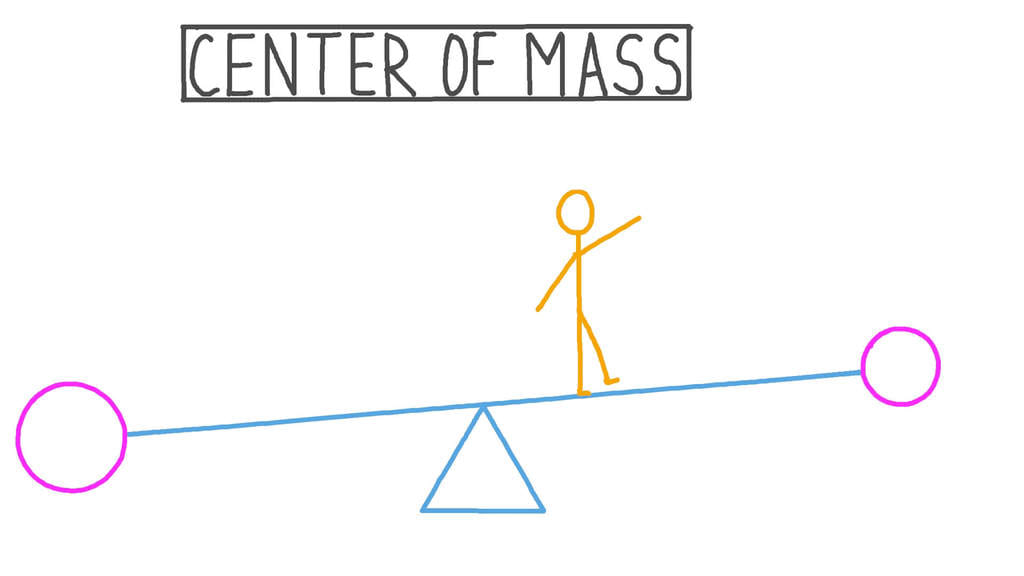Centre of Mass

• Every physical system has associated with it a certain point whose motion characterizes the motion of the whole system.
• When the system moves under some external forces, then this point moves as if the entire mass of the system is concentrated at this point and also the external force is applied at this point for translational motion. This point is called the centre of mass of the system.

Centre of Mass of a System of `N' Discrete Particles

Consider a system of N point masses m1, m2, m3, .................. mn whose position vectors from origin O are given by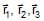........... respectively. Then the position vector of the centre of mass C of the system is given by: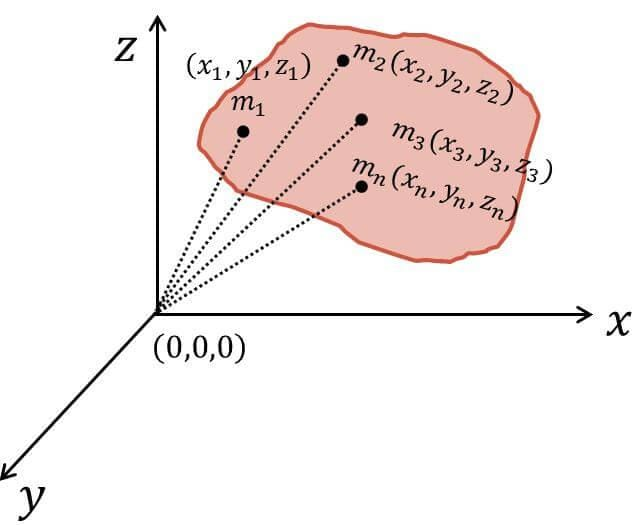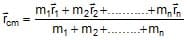;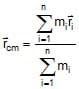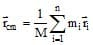where,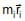is called the moment of mass of particle with respect to origin.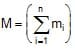is the total mass of the system.
Further,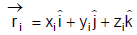and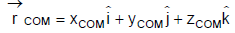So, the cartesian co-ordinates of the COM will be
xcom =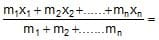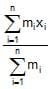or xCOM =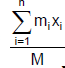Similarly, yCOM =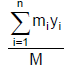and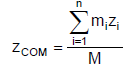Note:

• If the origin is taken at the centre of mass then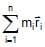= 0. hence, the COM is the point about which the sum of "mass moments" of the system is zero.
• If we change the origin then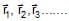changes. So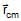also changes but exact location of center of mass does not change.

Position of COM of two Particles

Consider two particles of masses m1 and m2 separated by a distance l as shown in figure.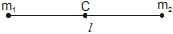Let us assume that m1 is placed at origin and m2 is placed at position (l, 0) and the distance of centre of mass from m1 & m2 is r1 & r2 respectively.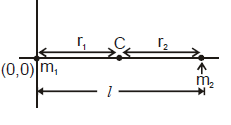So xCOM =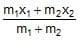r1 =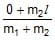=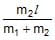...(1)
r2 =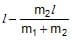=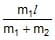...(2)
From the above discussion, we see that
r1 = r2 =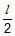if m1 = m2, i.e., COM lies midway between the two particles of equal masses.
Similarly, r1 > r2 if m1 < m2 and r1 < r2 if m2 < m1 i.e., COM is nearer to the particle having larger mass.
From equation (1) & (2),
m1r1 = m2r2 ...(3)
Centre of mass of two particle system lie on the line joining the centre of mass of two particle system.

Question for Centre of Mass
Try yourself:Can the centre of mass of the rigid body be located outside of the body?

Q.1. Two particle of mass 1 kg and 2 kg are located at x = 0 and x = 3 m. Find the position of their centre of mass.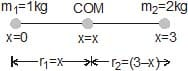Since, both the particles lie on x-axis, the COM will also lie on x-axis. Let the COM is located at x = x, then

r1 = distance of COM from the particle of mass 1 kg = x

and r2 = distance of COM from the particle of mass 2 kg = (3 -x)

Using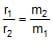or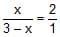or x = 2 m

thus, the COM of the two particles is located at x = 2m.

Q.2. Two particle of mass 4 kg & 2 kg are located as shown in figure then find out the position of centre of mass.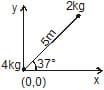First find out the position of 2 kg mass

x2kg = 5 cos 37° = 4 m

y2kg = 5 sin 37° = 3 m

So these system is like two particle system of mass 4 kg and 2kg are located (0, 0) and      (4, 3) respectively, then

xcom ==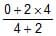=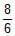=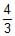ycom =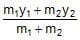=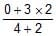= 1 m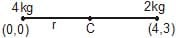So position of C.O.M isQ.3. Two particles of mass 2 kg and 4 kg lie on the same line. If 4 kg is displaced rightwards by 5 m then by what distance 2 kg should be move for which centre of mass will remain at the same position.

Let us assume that C.O.M. lie at point C and the distance of C from 2 kg and 4 kg particles are r1 & r2 respectively. Then from relation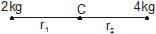m1r1 = m2r2

2r1 = 4r2                   ...(i)

Now 4 kg is displaced rightwards by 5 m then assume 2 kg is displaced leftwards by x distance to keep the C.O.M. at rest.

from relation m1r1 = m2r2'
⇒  m1(r1 + x) = m2 (r2 + y)
2(r1 + x) = 4(r2 + 5)    ...(ii)
2x = 20
x = 10 m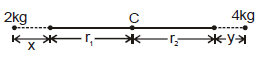To keep the C.O.M at rest 2 kg displaced 10 m left wards

Alter: If centre of mass is at rest then we can write

m1x = m2y

2 × x = 4 × 5

x = 10 m.

Q.4. Two particles of mass 1 kg and 2 kg lie on the same line. If 2 kg is displaced 10 m rightwards then by what distance 1 kg should displaced so that centre of mass will displaced 2m right wards.

Initially let us assume that C.O.M is at point C which is r1 & r2 distance apart from mass m1 & m2 respectively as shown in figure.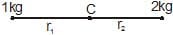from relation m1 r1 = m2 r2

⇒ (1) r1 = 2r2

Now 2kg is displaced 10 m rightwards then we assume that 1 kg is displaced x m leftward to move the C.O.M 2m rightwards.

So from relation m1r1' = m2r2'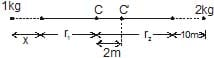⇒ 1 (x + r1 + 2) = 2 (10 + r2 - 2)
⇒ x + r1 + 2 = 20 + 2r2 - 4    ...(ii)

from eq. (i) & (ii) x = 14 m (leftwards).

Q.5. Three particles of mass 1 kg, 2 kg, and 3 kg are placed at the corners A, B and C respectively of an equilateral triangle ABC of edge 1 m. Find the distance of their centre of mass from A.

Assume that 1 kg mass is placed at origin as shown in figure.

co-ordinate of A = (0, 0)

co-ordinate of B = (1 cos60°,1 sin60°) =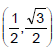co-ordinate of C = (1, 0)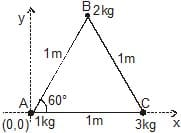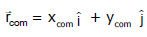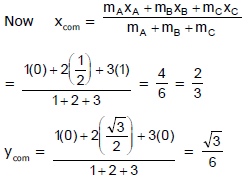Position of centre of mass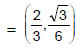distance of C.O.M from point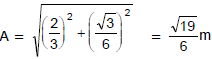Centre of Mass of a Continuous Mass Distribution

For continuous mass distribution the centre of mass can be located by replacing summation sign with an integral sign. Proper limits for the integral are chosen according to the situation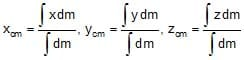...(i)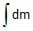= M (mass of the body)
here x, y, z in the numerator of the eq. (i) is the coordinate of the centre of mass of the dm mass.=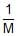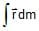Note :

• If an object has symmetric mass distribution about x axis then y coordinate of COM is zero and vice-versa.

Centre of Mass of a Uniform Rod

• Suppose a rod of mass M and length L is lying along the x-axis with its one end at x = 0 and the other at x = L. Mass per unit length of the rod l =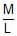• Hence, dm, (the mass of the element dx situated at x = x is) = l dx
• The coordinates of the element dx are (x, 0, 0). Therefore, x-coordinate of COM of the rod will be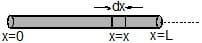xCOM =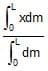=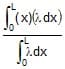=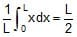• The y-coordinate of COM is
yCOM =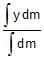= 0
• Similarly, zCOM = 0
i.e., the coordinates of COM of the rod are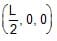, i.e, it lies at the centre of the rod.

Question for Centre of Mass
Try yourself:Does the explosion of a projectile in mid-air effects the motion of the centre of mass?

The document Centre of Mass Notes | Study Physics Class 11 - NEET is a part of the NEET Course Physics Class 11.
All you need of NEET at this link: NEET

## Physics Class 11

126 videos|449 docs|210 tests
 Use Code STAYHOME200 and get INR 200 additional OFF

## Physics Class 11

126 videos|449 docs|210 tests

### How to Prepare for NEET

Read our guide to prepare for NEET which is created by Toppers & the best Teachers

Track your progress, build streaks, highlight & save important lessons and more!

,

,

,

,

,

,

,

,

,

,

,

,

,

,

,

,

,

,

,

,

,

;